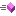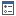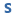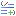VintaSoft Imaging .NET SDK v8.8 for .NET Framework
Vintasoft.Imaging Namespace / AffineMatrix Class
Properties Methods

In This Topic
AffineMatrix Class Members
In This Topic

The following tables list the members exposed by AffineMatrix.

Public Constructors
NameDescriptionOverloaded.
Top
Public Properties
NameDescriptionGets the determinant of the matrix.Gets a value that indicates whether this AffineMatrix class is an identity matrix.Gets a value that indicates whether the matrix is invertible.Gets or sets the value of the first row and first column of the matrix (scale X).Gets or sets the value of the first row and second column of the matrix.Gets or sets the value of the second row and first column of the matrix.Gets or sets the value of the second row and second column of the matrix (scale Y).Gets or sets the value of the third row and first column of the matrix (offset X).Gets or sets the value of the third row and second column of the matrix (offset Y).Gets the type of the matrix.
Top
Public Methods
NameDescriptionClones this instance of affine matrix.Overloaded. Creates a matrix, which is rotated relative point (0;0).Overloaded. Creates scaled matrix.Creates skewed matrix.Creates matrix and translates it to the specified offset.Overloaded. Compares two AffineMatrix.Returns the matrix elements.Returns the hash code for this instance.Returns an identity matrix.Overloaded. Inverts an AffineMatrix.Overloaded. Multiplies a AffineMatrix by another AffineMatrix.Multiplies the specified AffineMatrix to this AffineMatrix.Rotates this AffineMatrix about the specified point.Rotates this AffineMatrix about the specified point.Prepends a rotation of the specified angle at the specified point to this AffineMatrix.Prepends a rotation of the specified angle to this AffineMatrix.Appends the specified scale vector to this AffineMatrix.Scales this AffineMatrix by the specified amount about the specified point.Prepends the specified scale about the specified point of this AffineMatrix.Prepends the specified scale vector to this AffineMatrix.Changes this AffineMatrix into an identity matrix.Overloaded. Sets the current matrix form specified matrix.Appends a skew of the specified degrees in the x and y dimensions to this AffineMatrix.Prepends a skew of the specified degrees in the x and y dimensions to this AffineMatrix.Overloaded. Returns the string representation of this object.Appends a translation of the specified offsets to this AffineMatrix.Prepends a translation of the specified offsets to this AffineMatrix.Appends a translation of the specified X-axis offset to this AffineMatrix.Prepends a translation of the specified X-axis offset to this AffineMatrix.Appends a translation of the specified Y-axis offset to this AffineMatrix.Prepends a translation of the specified Y-axis offset to this AffineMatrix.
Top
Public OperatorsEquality Compares two AffineMatrix.Explicit Type Conversion Performs an explicit conversion from AffineMatrix to System.Drawing.Drawing2D.Matrix.Implicit Type Conversion Performs an implicit conversion from System.Drawing.Drawing2D.Matrix to AffineMatrix.Inequality Compares two AffineMatrix.Multiplication Multiplies a AffineMatrix by another AffineMatrix.
Top
See Also